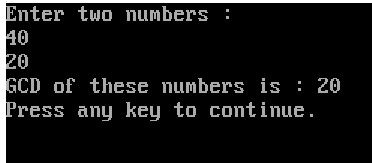# Write a program in C++ to find the Greatest Common Divisor (G.C.D.) of two natural numbers.

more_vert

Write a program in C++ to find the Greatest Common Divisor (G.C.D.) of two natural numbers.

more_vert

verified//Program to find the G.C.D. of two numbers

#include<iostream.h>
#include<conio.h>
int gcd(int a, int b);
void main()
{
int x, y, g;
clrscr();
cout<<"Enter two numbers : "<<endl;
cin>>x>>y;
g=gcd(x,y);
cout<<"GCD of these numbers is : "<<g;
getch();
}

int gcd(int a, int b)
{
int r;
r=a%b;
while (r>0)
{
a=b;
b=r;
r=a%b;
}
return b;
}